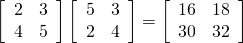# Machine Learning Part 6 — Matrix multiplication in SQL

This is the sixth part of the ML series. For your convenience you can find other parts in the table of contents in Part 1 – Linear regression in MXNet

Today we are going to implement a matrix multiplication in Redshift. Let’s go.

First, let’s see what we want to calculate:Nothing fancy. We would like our algorithm to be extensible to any sizes and non-uniform matrices as well.

We store the matrices as a rows where each row represents one value for given row and column. Rows and columns are one-based.

First, we need to calculate size of the result:

So we just get the maximum width and maximum height from the respective matrices. Now, we want to generate all the cells we need to fill:

So we basically do the Cartesian product and we are done. Now, we would like to get correct pairs for each cell:

This is what we get for our sample matrices:

Looks good. Now we just need to aggregate the pairs:

And we are done. You can see the code here.